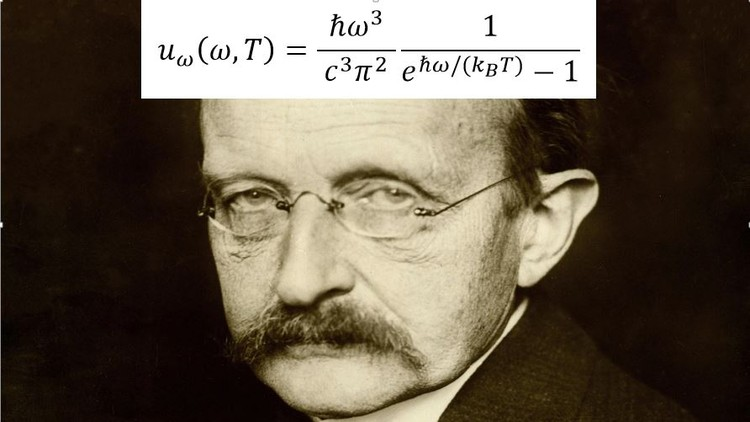All Courses

# The Mathematical Beauty that led to Quantum Physics## The Mathematical Beauty that led to Quantum Physics

### The Rise of Quantum Physics

#### What you’ll learn

The Mathematical Beauty that led to Quantum Physics

• mathematical tricks
• physical insights
• history of physics
• Basics of Statistical Mechanics

#### Requirements

• Calculus (especially derivatives, integrals, limits)
• Multivariable Calculus
• Basics of Fourier Analysis

#### Description

This course showcases the beautiful mathematics that, in the late 19th century/ early 20th century, led to the discovery of a revolutionary branch in physics: Quantum Mechanics.

Planck postulated that the energy of oscillators in a black body is quantized. This postulate was introduced by Max Planck in the derivation of his law of black body radiation in 1900. This assumption allowed Planck to derive a formula for the entire spectrum of the radiation emitted by a black body (we will also derive this spectrum in this course). Planck was unable to justify this assumption based on classical physics; he considered quantization as being purely a mathematical trick, rather than (as is now known) a fundamental change in the understanding of the world.

Calculus and Multivariable Calculus are a prerequisite to the course; other important mathematical tools (such as Fourier Series, Parseval’s theorem, binomial coefficients, etc.) will be recalled, with the emphasis being put on mathematical and physical insights rather than abstract rigour.

#### Who this course is for:

• Students with a strong interest in mathematics and its beauty
• Students who would like to improve their reasoning and insights in solving physical problems
• The Students interested in the historical fascinating origin of Quantum Physics
• Students interested in explanations given through the lens of mathematics
• Last updated 7/2021

Content From: https://www.udemy.com/course/the-mathematical-beauty-that-led-to-quantum-physics/

Get More Courses FreeCourseSite